Next: 8.3 Spirals
Up: 8 Special Plane Curves
Previous: 8.1 Algebraic Curves

# 8.2 Roulettes (Spirograph Curves)

Suppose given a fixed curve C and a moving curve M, which rolls on C without slipping. The curve drawn by a point P kept fixed with respect to M is called a roulette, of which P is the pole.

The most important examples of roulettes arise when M is a circle and C is a straight line or a circle, but an interesting additional example is provided by the catenary y=a cosh(x/a), which arises by rolling the parabola y=x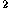/(4a) on the x-axis with pole the focus of the parabola (that is, P=(0,a) in the initial position). The catenary is the shape taken under the action of gravity by a chain or string of uniform density whose ends are held in the air.

A circle rolling on a straight line gives a trochoid, with the cycloid as a special case when the pole P lies on the circle (Figure 1). If the moving circle M has radius a and the distance from the pole P to the center of M is k, the trochoid has the parametric equation

x=a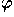-k sin, y=a-k cos.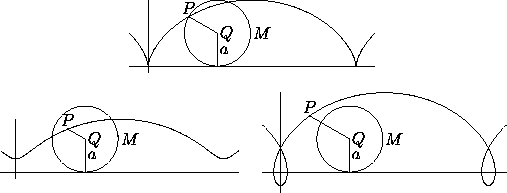Figure 1: Cycloid (top) and trochoids with k=.5a and k=1.5a, where k is the distance PQ from the center of the rolling circle to the pole.

The cycloid, therefore, has parametric equation

x=a(- sin),
y=a(1- cos).

One can eliminateto get x as a (multivalued) function of y, which takes the following form for the cycloid:The length of one arch of the cycloid is 8a, and the area under the arch is 3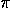a.

A trochoid is also called a curtate cycloid when k<a (that is, when P is inside the circle) and a prolate cycloid when k>a.

A circle rolling on another circle and exterior to it gives an epitrochoid. If a is the radius of the fixed circle, b that of the rolling circle and k is the distance from P to the center of the rolling circle, the parametric equation of the epitrochoid is

x=(a+b) cos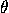- k cos ((1+a/b)),
y=(a+b) sin- k sin ((1+a/b)).

These equations assume that at the start everything is aligned along the positive x-axis, as in Figure 2, left.Figure 2: Left: Initial configuration for epicycloid (black) and configuration at parameter value(red). Middle: epicycloid with ba (nephroid). Right: epicycloid with b=a (cardioid).

Usually one considers the case when a/b is a rational number, say a/b=p/q where p and q are relatively prime. Then the rolling circle returns to its original position after rotating q times around the fixed circle, and the epitrochoid is a closed curve---in fact, an algebraic curve. One also usually takes k=b, so that P lies on the rolling circle; the curve in this case is called an epicycloid. The middle diagram in Figure 2 shows the case b=ka, called the nephroid; this curve is the cross section of the caustic of a spherical mirror. The diagram on the right shows the case b=k=a,   which gives the cardioid (compare Figure 8.1.6 , middle).

Hypotrochoids and hypocycloids are defined in the same way as epitrochoids and epicycloids, but the rolling circle is inside the fixed one. The parametric equation of the hypotrochoid is

x=(a-b) cos+ k cos ((a/b-1)),
y=(a-b) sin- k sin ((a/b-1)),

where the letters have the same meaning as for the epitrochoid. Usually one takes a/b rational and k=b. There are several interesting particular cases:

• b=k=a gives a point.
• b=ka gives a diameter of the circle C.
• b=k=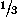a gives the deltoid (Figure 3, left), which has the algebraic equation

(x+y)-8ax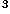+24axy+18a(x+y)-27a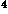=0.

• b=ka gives the astroid (Figure 3, right), an algebraic curve of degree six whose equation can be reduced to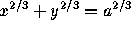. The figure illustrates another property of the astroid: its tangent intersects the coordinate axes at points that are always the same distance a apart. Otherwise said, the astroid is the envelope of a moving segment of fixed length whose endpoints are constrained to lie on the two coordinate axes.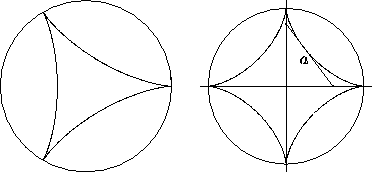Figure 3: The hypocycloids with a=3b (deltoid) and a=4b (astroid).

Next: 8.3 Spirals
Up: 8 Special Plane Curves
Previous: 8.1 Algebraic CurvesThe Geometry Center Home Page

Silvio Levy
Wed Oct 4 16:41:25 PDT 1995

This document is excerpted from the 30th Edition of the CRC Standard Mathematical Tables and Formulas (CRC Press). Unauthorized duplication is forbidden.